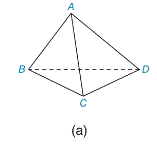Chapter 9.4, Problem 2EElementary Geometry For College St...

7th Edition
Alexander + 2 others
ISBN: 9781337614085

Solutions

Chapter
SectionElementary Geometry For College St...

7th Edition
Alexander + 2 others
ISBN: 9781337614085
Textbook ProblemFor Figure (a) of Exercise 1, find the number of faces, vertices, and edges in the polyhedron. Then verify Euler’s equation for that polyhedron.

To determine

To find:

The number of faces, vertices and edges in the polyhedron and verify Euler’s equation.

Explanation

Approach:

A) Polyhedron

1) A polygon is a two dimensional shape form with more than two straight lines.

2) A polyhedron is a three-dimensional solid shape.

3) Each flat surface of a polyhedron is a polygon and is called a face.

4) The line segment where two faces of a polyhedron meet is called an edge.

5) The point where three or more edges of a polyhedron meet is called a vertex.

B) Euler’s Equation

A very important relationship between the number of vertices, faces and edges of solid shapes was discovered by a Swiss mathematician Leonard Euler.

It states V+FE=2.

Where, V=Number of vertices, F=number of faces, E=number of edges.

Calculation:

The polyhedron ABCD is a triangular pyramid, also called as a tetrahedron

Still sussing out bartleby?

Check out a sample textbook solution.

See a sample solution

The Solution to Your Study Problems

Bartleby provides explanations to thousands of textbook problems written by our experts, many with advanced degrees!

Get Started

Evaluate dy if y = x3 2x2 + 1, x = 2, and dx = 0.2.

Single Variable Calculus: Early Transcendentals, Volume I

Simplify: 2736

Elementary Technical Mathematics

In problems 15-26, evaluate each expression. 24.

Mathematical Applications for the Management, Life, and Social Sciences